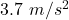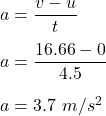## A car accelerates uniformly from 0 km/hr to 60 km/hr in 4.5 seconds. Which one of the following choices best represents the acceleration of

Question

A car accelerates uniformly from 0 km/hr to 60 km/hr in 4.5 seconds. Which one of the following choices best represents the acceleration of the car?
(A) 13.3 m/s2
(B) 9.8 m/s2
(C) 4.8 m/s2
(D) 3.7 m/s2
(E) 0.37 m/s2

in progress 0
5 months 2021-08-31T10:10:56+00:00 1 Answers 2 views 0

The acceleration of the car is.

Explanation:

It is given that,

Initial speed of the car, u = 0

Final speed of the car, v = 60 km/h = 16.66 m/s

Time, t = 4.5 s

We need to find the acceleration of the car. It is equal to the change in velocity divided by time taken. It can be given by :So, the acceleration of the car is. Hence, this is the required solution.